Maths
Study Material

# Determinants Class 12

Determinants are used in linear algebra and calculus. Calculating the determinants of square matrices of order less than 3 is easier. Let us discuss the ways of calculating them.

5 minutes long
Posted by Tanwir Silar, 1/9/2021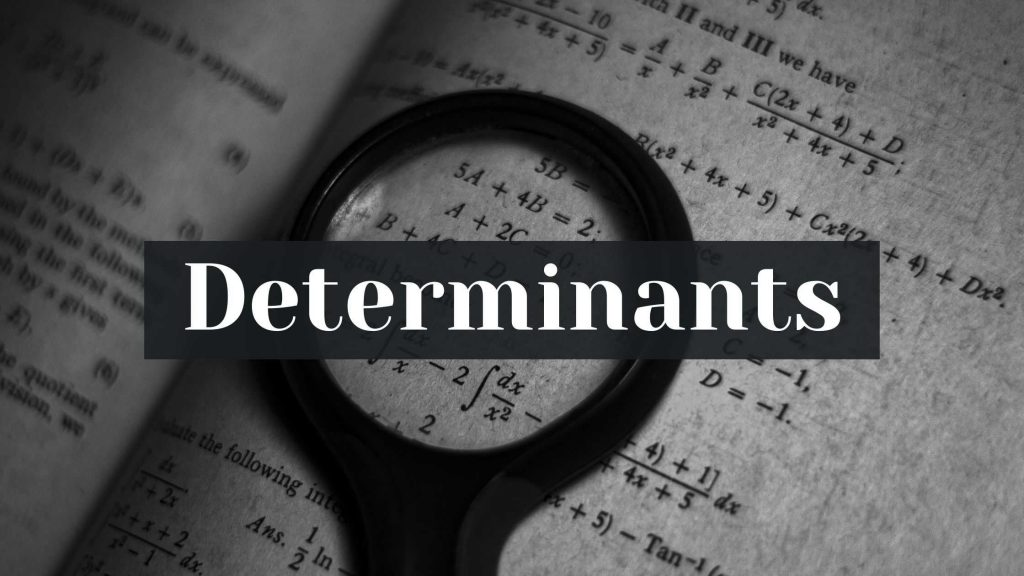Hesap Oluştur

Got stuck on homework? Get your step-by-step solutions from real tutors in minutes! 24/7. Unlimited.

The concept, determinant of a matrix, is key to solve myriad problems in mathematics, which include finding the inverse of a matrix, solving system of linear equations, finding the area of a triangle, finding if three points are collinear or not and determinants also used in calculus and linear algebra. We will read more here about determinants class 12.

## What are determinants?

Determinants are unique values associated with matrices. Determinants exist for every square matrix A = [aij] of order n. They are represented in two ways, either as det(A) or |A|. We can calculate the determinant of a matrix of order n using expansion along any row or any column and corresponding minors. Usually, it is calculated by expanding along the first row with the use of their minors.

## Finding the determinant of matrices

We are going to discuss the ways to find the determinant for matrices with order less than or equal to 3.

#### Determinant of a matrix of order 1:

Any matrix of order 1 has one and only one element and the determinant of such matrix is equal to the value of that element.
For instance, let A = , be a 1×1 matrix, the value of its determinant is equal to 7.

#### Determinant of a matrix of order 2:

Let A =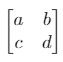, the determinant of such a matrix is equals to ad-bc.
We can write det(A) = ad-bc = |A|, sometimes it is also written as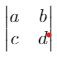= ad-bc.

#### Determinant of a matrix of order 3:

To be able to calculate the determinant of a matrix of order 3, we need to know the concept of minors.
Each elements in a square matrix has its own minor. The determinant of the matrix obtained by deleting the row and column of the element under consideration is the minor the element. Let us look through few examples.
For the matrix A =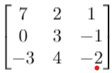,
the minor of the element 7 is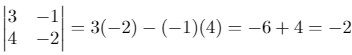the minor of the element 3 is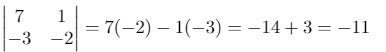The determinant of a matrix when expanded along the column j is equal to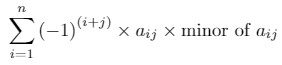when expanded along the column i is equal to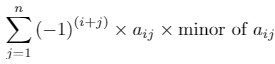Now, let us try to find the determinant of a 3×3 matrix. As discussed earlier, the determinant of a matrix can be calculated by expanding along any row or column. Let us look at one of the ways by expanding along the first row.

The elements in the first row are 7, 2, 1.
The minor of 7 isAlso, The minor of 2 is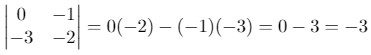The minor of 1 is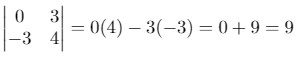Using the formulato calculate the determinant, we get
det(A) is equals to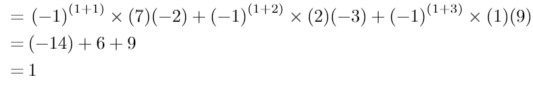So, the determinant of the matrix A is 1. The same value is got by expanding along any row or along any column, since the determinant of a matrix is unique.

We hope, this blog(Determinants class 12) was helpful.